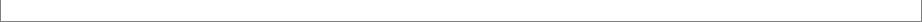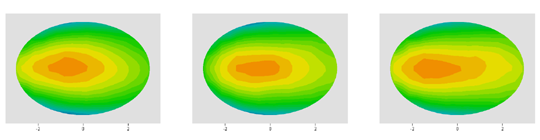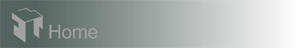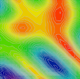Case 19: Material Budgeting - Parabolic Mirror

In this example we show how V-Grade 5 can help in material budgeting.

Suppose that the job is to gold coat parabolic mirrors in the planetary-rotation coater that we modeled in the previous cases with a pair of uncoupled masks. We consider parabolic mirrors, shown in the drawing below, with a reflective surface that is 5 cm in its width and approximately 6.8 cm in its length.
We can place three of these mirrors, lengthwise, along a radius of the fixture (25 cm). We first position the mirrors in such a way that the two end points in the length direction are leveled with the radius of the fixture. A simulation run immediately exposes that this arrangement yields a poor thickness distribution: the thickness distribution is significantly lopsided - thinner towards the apex of the parabola.

To improve the thickness distribution we can pivot the mirrors. After a few trials and errors we arrive at an arrangement shown in the following fixture views. The three mirrors are pivoted by 2.8 degrees, 6.8 degrees, and 12.8 degrees, respectively.The pivoted mirrors yields much improved thickness distributions, shown in the contour plots below. The layers deposited on the three mirrors have very similar average thickness with an overall peak-to-valley nonuniformity of 1.6%.
max=24.46; min=23.71
max=24.47; min=23.72
max=24.47; min=23.78
Recall that V-Grade 5 computes and yields thicknesses in units of "nanometer per gram of source material evaporated", we can now know how much gold we need for a desired average thickness of 1000 angstroms. By entering, In the System & Material panel, the density of gold of 19.3, the resultant average thickness 24.2 is in units of "nanometers per gram of gold evaporated". This tells us that each coating run in this coater consumes 4.13 grams of gold.

If we employ the technique of terracing, instead of correction masks, we can obtain very similar thickness distribution in and among the mirrors with an average thickness of approximately 27 nm per gram of gold. For the same 1000-angstrom coating the consumption of gold would be 3.7 grams.Hardware requirements
Request a Free Trial